# Stacked Bar Chart

A stacked bar chart is a type of graph that displays multiple categories of data as bars stacked on top of each other. Each bar segment represents a portion of the whole, and the height of the bar represents the total of all segments. Use stacked bar chart for a holistic view of data breakdown.Transcript# Creating a Stacked Bar Chart widget

• Under any App, go to reports
• Click + Report
• Select the list you want to get the data from.
• From the Chart Type, select Stacked Bar Chart
• Then continue to configure it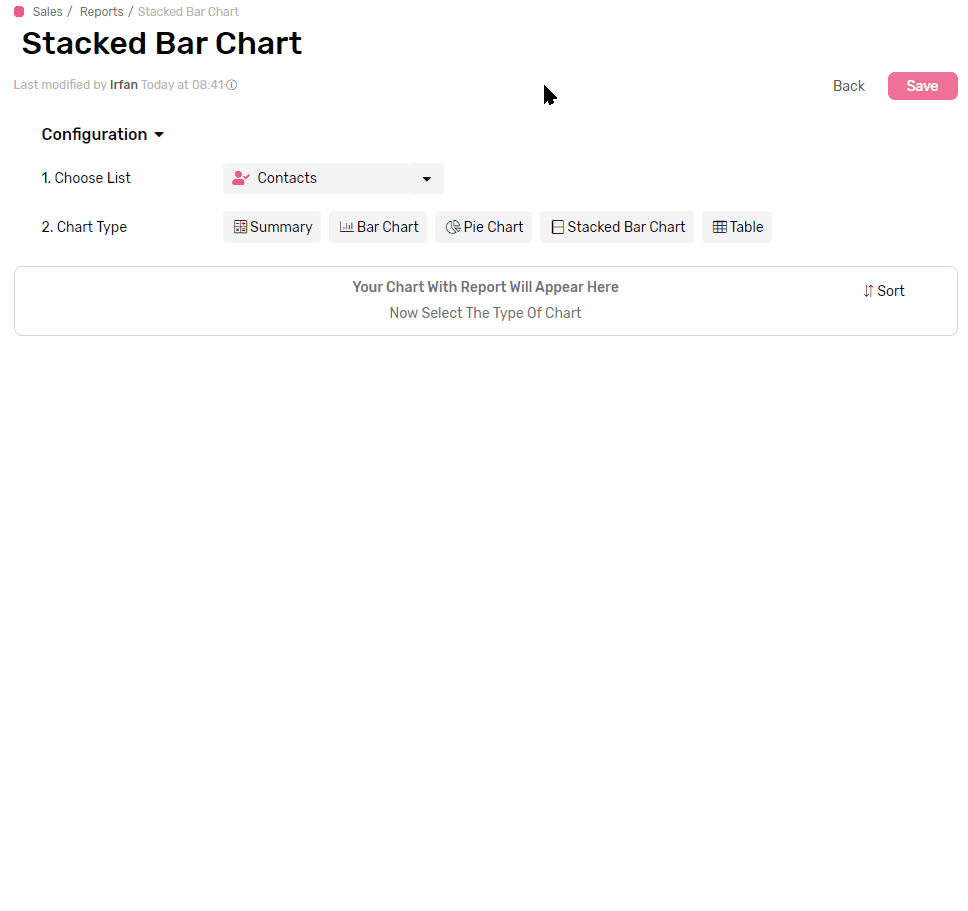## Functions

Applying a mathematical function to all values in each group, e.g. get a total of all values, applying a filter later in the configuration will limit the function to work only on the values from records that match the filter.

• Sum will calculate the total of all values in each group.
• Average will calculate the mathematical average of all values in each group.
• Max will retrieve the Maximum value in each group
• Min will retrieve the Minimum value in each group
• Count will count the records which have a value in the selected field
• Count is the only function that accepts all types of fields.
•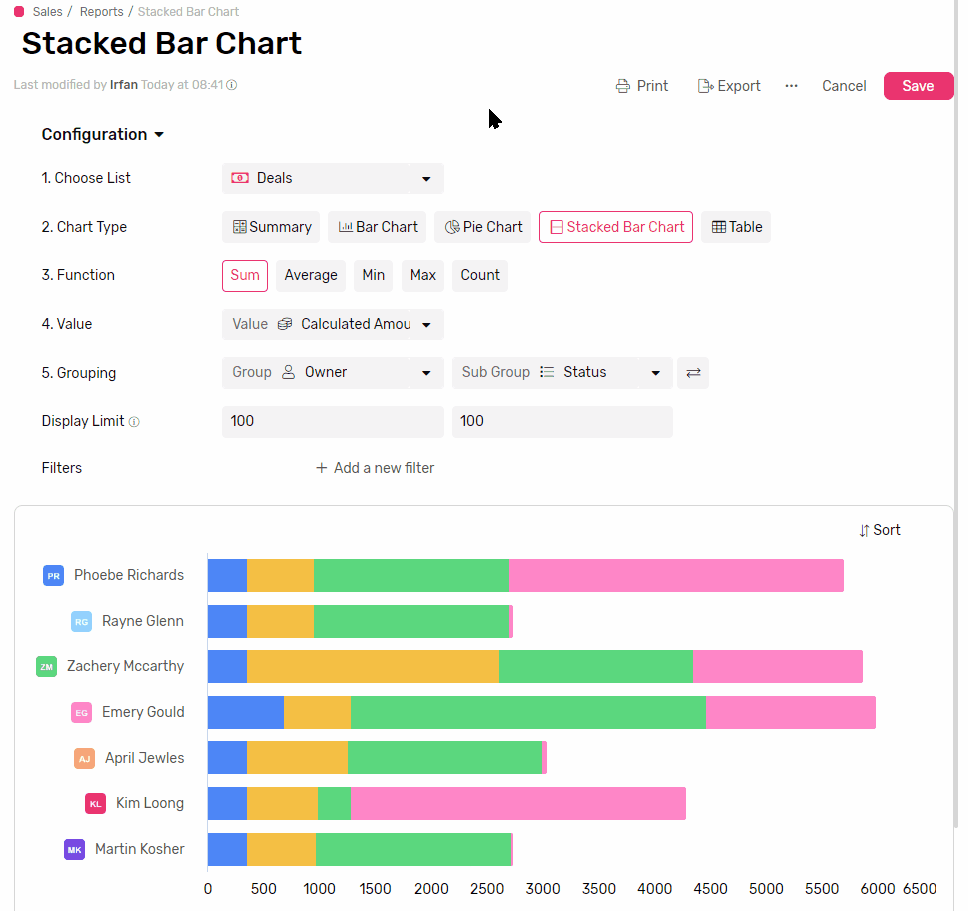## Value

• This is where you select the field that you want the function to run on.

## Grouping

### Main Group (Bars)

• This is the mandatory grouping field, that you can’t create a stacked Bar chart without.
• Select a field to group the data by, each group will be represented by a bar.
• If you group by a date field you can also set the frequency
• Frequency can be Daily, Weekly, Monthly, or Yearly
• Each bar will represent a time frame determined by the frequency (e.g. if you select weekly, each bar will represent a week.)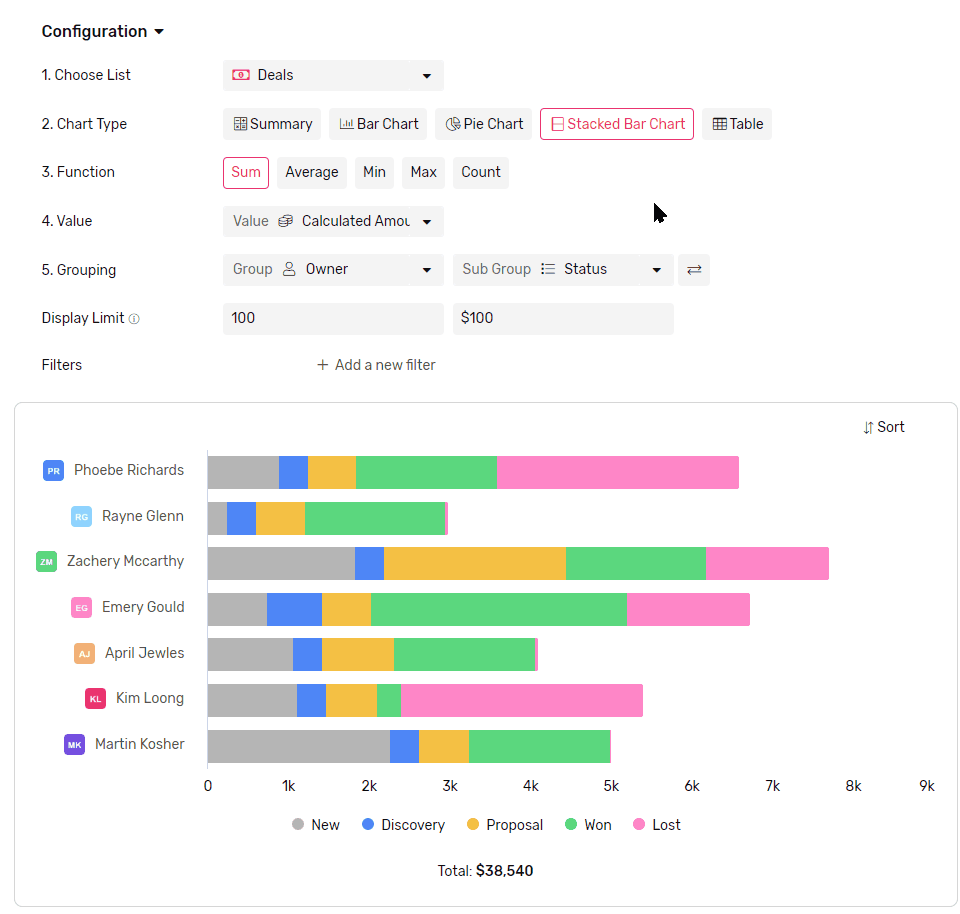### Secondary Group (Stack)

• If you select a secondary group, they will show up as Stacks to the stacked Bar
• Each stack will represent a subset of data from the main group represented by the bar.

### Switch grouping

• You can easily switch Stacks and Bars by clicking the switch button right next to them.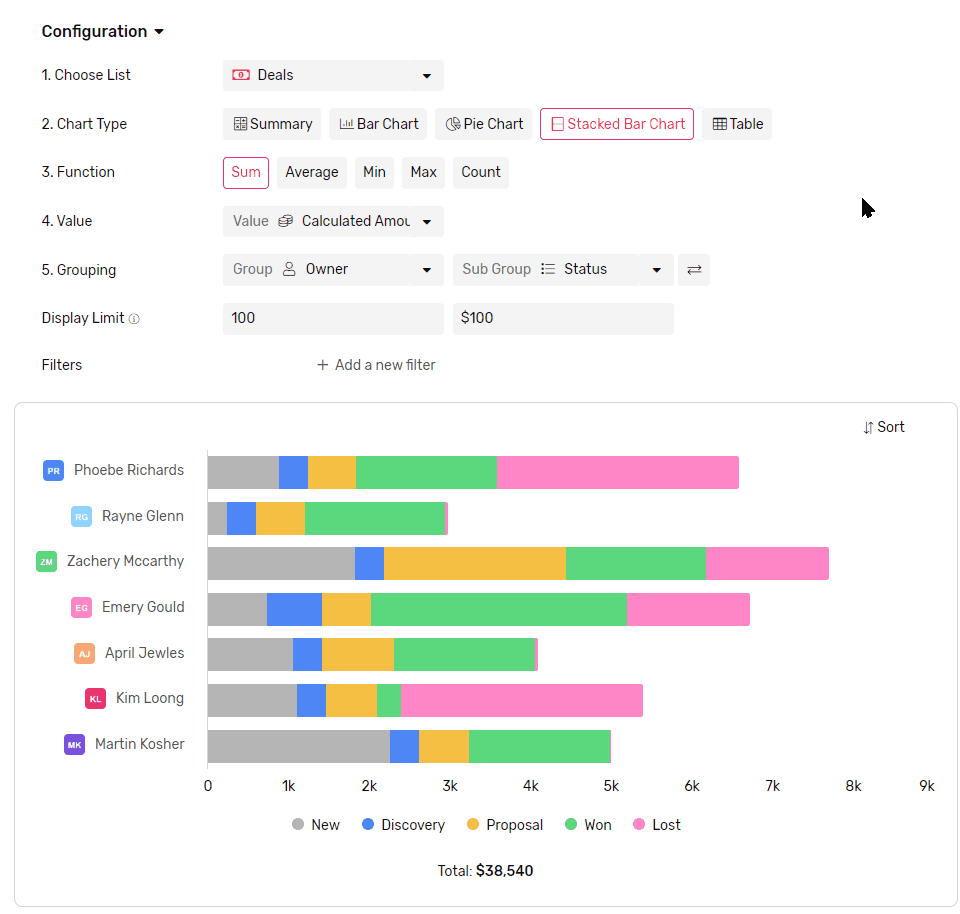## Display Limit

• Limit how many bars are displayed in the chart.
• The maximum number of bars you can see in the chart at a time is 100.
• If there are more groups than the limit the rest of the groups will be all gathered under one bar called others.
• To better control which bars are showing you can use a mix of Filters and Sorting.
• The same applies to Stacks as well, with the sub-grouping limit.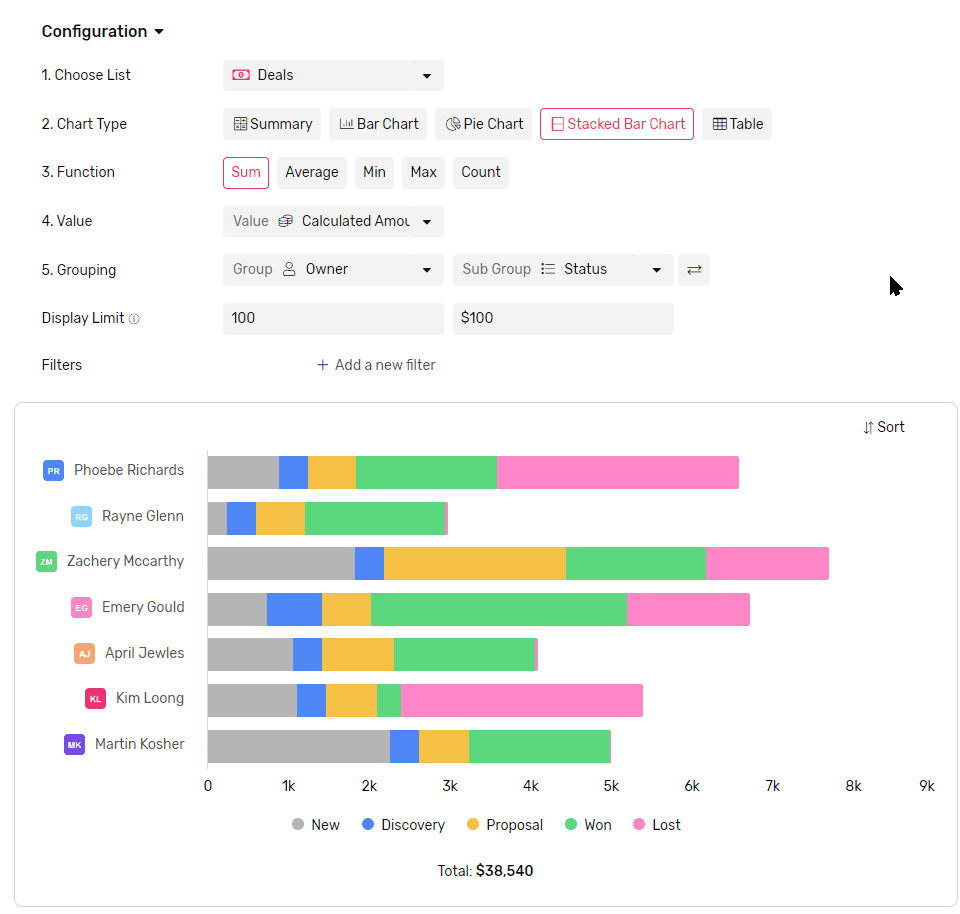## Sorting

• You can sort your bars either by
• Value, which will sort based on comparing the value represented in the bars.
• Legend, which will sort based on bars labels.
• The same applies to stacks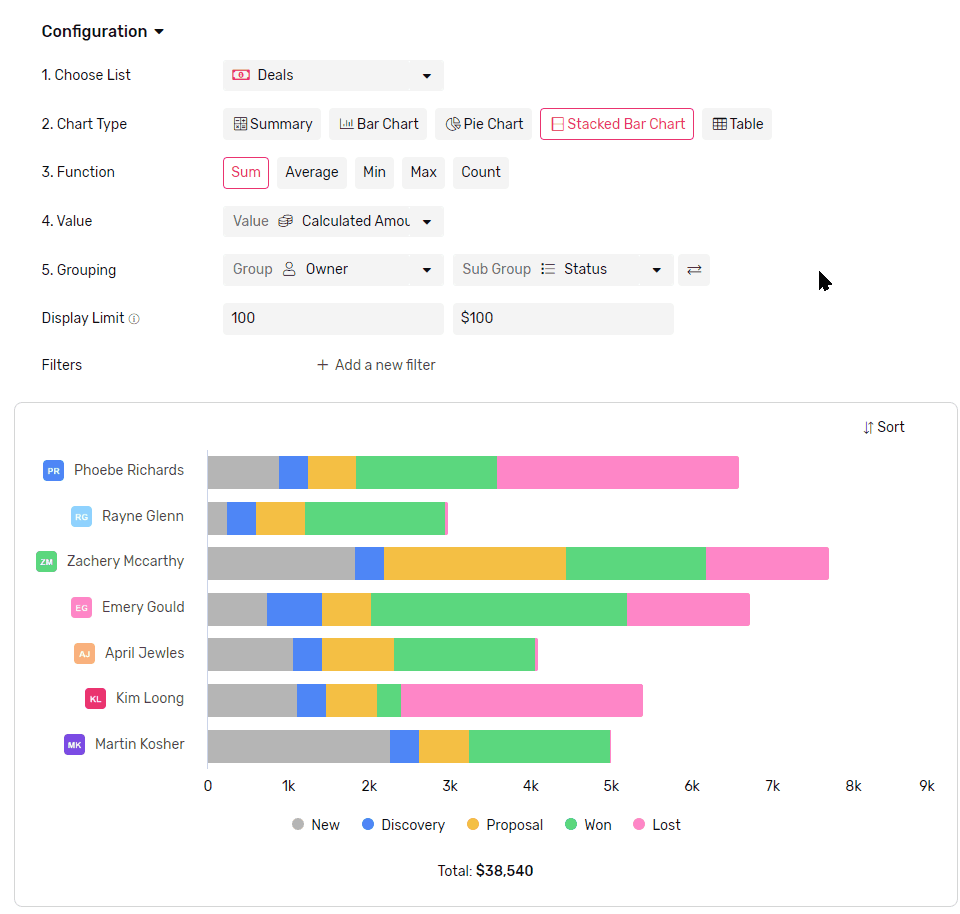### Looking for something else?

Search by entering some keywords such as; 'email automation', 'linked list'...